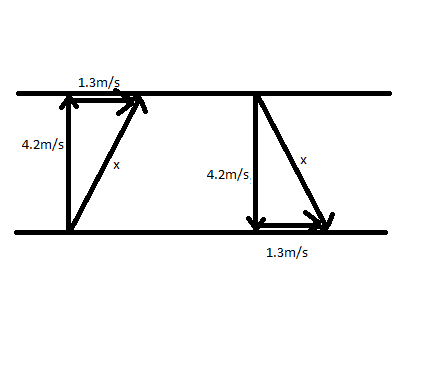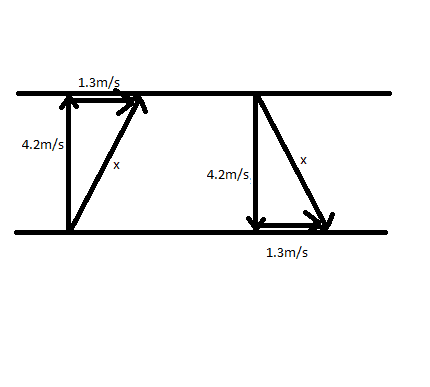# Swimming Across River Question

physicsnobrain

## Homework Statement

A man can swim through still water 4.2m/s. Suppose this man swims in a river with current 1.3 m/s. Find his average speed over a trip:
a) across the river and back
b) a certain distance downstream and back

## The Attempt at a Solution

a)
Here is a diagram I made:Left side of picture is there, right side is back

So should I solve for x, then take x, multiply by two, then divide by two. Thats the avg speed? because the question doesnt give any distance or time, so this is all I could think of.

b) is this a vector addition question? so his avg speed would be 2.9m/s?

Thanks

Homework Helper
Gold Member
a. Avg speed is x, right.
b. Careful with this one. Remember, avg speed = total distance/total time. Does not equal 2.9.

physicsnobrain
a. Avg speed is x, right.
b. Careful with this one. Remember, avg speed = total distance/total time. Does not equal 2.9.

So can I choose any distance? Since I am not given one.

Homework Helper
Gold Member
So can I choose any distance? Since I am not given one.

Right.

physicsnobrain
Ok. I chose 16m as my distance downstream.

After computing it I get 4.1m/s as the avg speed for the trip

Homework Helper
Gold Member
Not what i got. Show your work?

physicsnobrain
ok first i add the vectors. On the downstream his velocity increases to 7.1m/s while the upstream is 2.9 m/s.

Using these two found values i calculate time for the 16m upstream and 16m downstream. using t = d/v

For upstream I get 5.52s and downstream i get 2.25s.

Now I do avg spd. total distance/total time. I add up the distances i get 32m, i add up the times i get 7.77s. 32/7.77s I get 4.12m/s

is this not correct?

Homework Helper
Gold Member
ok first i add the vectors. On the downstream his velocity increases to 7.1m/s while the upstream is 2.9 m/s.

???

physicsnobrain
ok first i add the vectors. On the downstream his velocity increases to 7.1m/s while the upstream is 2.9 m/s.

???

He swims 4.2m/s through still water. There is a current of 1.3m/s. When hes downstream he goes faster, you have to add them to get 7.1m/s, when upstream you subtract the current from his speed.

The question asks about him swimming directly into the current. He goes straight, not across.

Homework Helper
Gold Member
He swims 4.2m/s through still water. There is a current of 1.3m/s. When hes downstream he goes faster, you have to add them to get 7.1m/s, when upstream you subtract the current from his speed.

The question asks about him swimming directly into the current. He goes straight, not across.

OK but where I come from, 4.2 + 1.3 does not equal 7.1.

skeptic2

## Homework Statement

A man can swim through still water 4.2m/s. Suppose this man swims in a river with current 1.3 m/s. Find his average speed over a trip:
a) across the river and back

Here is a diagram I made:Left side of picture is there, right side is back

So should I solve for x, then take x, multiply by two, then divide by two. Thats the avg speed? because the question doesnt give any distance or time, so this is all I could think of.

As I read the problem, "across the river and back" to me means that he ends up at his starting point. Your diagram indicates he would end up 2.6 downstream. In order for him to arrive back at his starting point you would have to interchange the 4.2 m/s and x on your diagram.

physicsnobrain
OK but where I come from, 4.2 + 1.3 does not equal 7.1.

Oops silly error. Now with the correct numbers I get an avg spd of 3.8m/s

physicsnobrain
As I read the problem, "across the river and back" to me means that he ends up at his starting point. Your diagram indicates he would end up 2.6 downstream. In order for him to arrive back at his starting point you would have to interchange the 4.2 m/s and x on your diagram.

yes you are right. I now get 4.2m/s as the penguins average speed for part a

physicsnobrain
yes you are right. I now get 4.2m/s as the penguins average speed for part a

Is this number correct?

Homework Helper
Gold Member
Oops silly error. Now with the correct numbers I get an avg spd of 3.8m/s

That's what I got.

skeptic2
How did you get 3.8 m/s? Can you show your work? I get a value slightly higher.

What did you get for part b?

physicsnobrain
How did you get 3.8 m/s? Can you show your work? I get a value slightly higher.

What did you get for part b?

I got 3.8 for part b. For part a I got 4.2m/s.

Homework Helper
Gold Member
How did you get 3.8 m/s? Can you show your work? I get a value slightly higher.

What did you get for part b?

That was part b. Same as what you got.

I never answered part a but 4.2 looks wrong. 4.2 is his speed in calm water. I think the answer is the same as what you would have gotten in your original post: x^2 = 4.2^2 + 1.3^2.

physicsnobrain
That was part b. Same as what you got.

I never answered part a but 4.2 looks wrong. 4.2 is his speed in calm water. I think the answer is the same as what you would have gotten in your original post: x^2 = 4.2^2 + 1.3^2.

Are you sure? I think skeptic may have a point about having to return to the same starting point.

Homework Helper
Gold Member
Yes, but I think the answer's the same. He would start upstream by a certain distance and wind up at the starting point, and the velocity vectors would be the same as if he wound up downstream by the same distance.

physicsnobrain
Yes, but I think the answer's the same. He would start upstream by a certain distance and wind up at the starting point, and the velocity vectors would be the same as if he wound up downstream by the same distance.

Ok, well then in this case the avg speed is 4.4m/s

Homework Helper
Gold Member
Ok, well then in this case the avg speed is 4.4m/s

Agreed.

skeptic2
I'm sorry, I think my post #16 was a little mixed up. I get the same answer as you, 3.8 m/s.